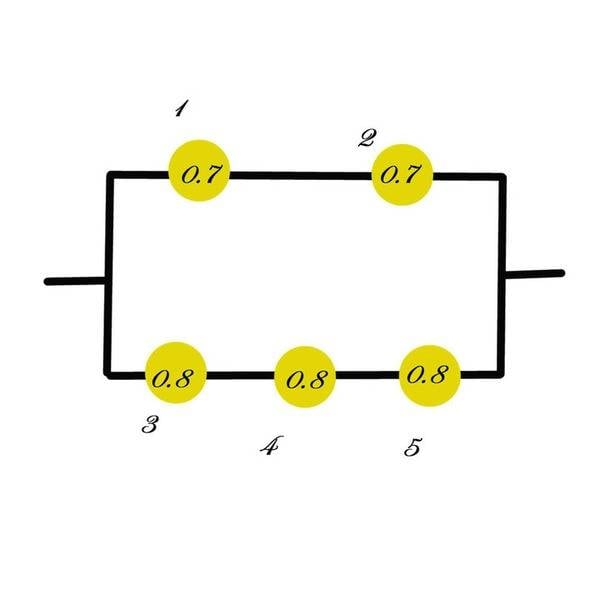# Probability questionThe numbers represent the probability that each component works.

a) What's the probability that the entire system works?
b) Given that the system works, what's the probability that the component A is not working?

a) P=.75112, easy
b) Don't know what to do here....

Homework Helper
Since there is no component A in the diagram, I'd say you can't answer the second question.
If you were asking this: Given the system works, what is the probability $$1$$
is not working, think this way:

If the system is operating, you know that
* $$3, 4$$ and $$5$$ are all operating
and
* $$1$$ is not operating, status of $$2$$ is unimportant

what can you do with this?